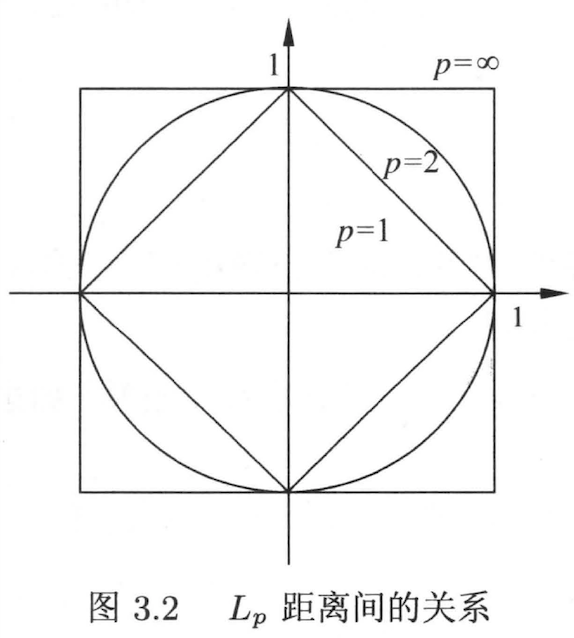# 机器学习笔记-KNN（统计学习方法-第3章）

## 2020-10-07

K近邻（KNN）是一种基本分类与回归方法。给定目标样本通过K个邻居来决定目标样本的标签。K近邻法有三个基本要素：K值的选择、距离度量和分类回归决策规则。KNN并不需要训练，但需要遍历整个训练集，所以预测比较慢，书中提到用KD树进行优化，来提高K近邻搜索的效率。

## K近邻基本要素

### 距离度量

KNN一般采用欧式距离，但更一般的是 $L_p$ 距离， $L_p$ 距离在机器学习中经常会看到。

$p = 1$ 时，称为曼哈顿距离，即 $L_1(x_i, x_j) = \sum_{l=1}^n |x_i^l - x_j^l|$

$p = 2$ 时，称为欧式距离，即 $L_2(x_i, x_j) = (\sum_{l=1}^n |x_i^l - x_j^l|^2) ^{\frac{1}{2}}$ ，写成我们最熟悉的形式 $\sqrt{(\Delta x_1)^2 + (\Delta x_2)^2 + ... + (\Delta x_n)^2}$

$p = \infty$ ，它是各个坐标距离的最大值，即 $L_\infty(x_i, x_j) = \underset{l}{max} \ |x_i^l - x_j^l|$### K值选择和决策

K值选择会对KNN的结果产生很大影响。K既不能太小也不能太大。如果选择较小的 $K$ 值，极端取 $K=1$ 就意味着模型变得复杂，容易发生过拟合；如果选择较大的 $K$ 值，极端取 $K = N$$N$ 即为样本的数量），那么模型过于简单（容易欠拟合）。书上用了近似误差（关注的是训练集）和估计误差（关注的是测试集即泛化性）来描述的，知乎上有关于近似误差和估计误差的详细解释（链接）。

KNN在分类问题中往往是多数表决，即由输入实例的K个邻近的训练实例中的多数类决定最终结果；

KNN在回归问题中是取平均，即对样本的K个邻近标签值取平均，作为预测结果。

## KD树

K近邻算法如果用线性扫描，则时间复杂度为 O(N)，当训练集很大时，计算非常耗时；所以为了提高K近邻搜索效率，使用特殊的存储结构（即KD树），以减少扫描的次数，时间复杂度为 O(logN)；可以理解为用空间换时间，空间体现在存储结构的构建，这种“空间换时间”的思想在机算机科学里屡见不鲜。

KD树需要 先构造，再搜索，注意这里的 “KD树的K” 和 “K近邻的K” 含义不同，这里的K是指数据的维度。

## 总结

KNN的理论比较通俗易懂，后续有空可以尝试些代码的实践，KNN的包在sklearn里有集成（ 链接 ）。

## 参考文献

1. l1正则与l2正则的特点是什么，各有什么优势？ ，知乎
2. 《统计学习方法》第3章-K近邻法，李航
3. 统计学习方法-K近邻算法 ，CSDN